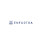# UGC NET Computer Science Questions Paper II December 2008 - Part 3

21.       N processes are waiting for I/O. A process spends a fraction p of its time in I/O wait state. The CPU utilization is given by:
(A) 1-p-N
(B) 1-pN
(C) pN
(D) p-N
Explanation:
According to the probabilistic model, if a process spends a fraction p of its time in I/O wait state, and if there are N processes in memory, then the CPU utilization is given by 1-pN
22.       If holes are half as large as processes, the fraction of memory wasted in holes is:
(A) 2/3
(B) 1/2
(C) 1/3
(D) 1/5
23.       An example of a non pre-emptive scheduling algorithm is:
(A) Round Robin
(B) Priority Scheduling
(C) Shortest job first
(D) 2 level scheduling
24.       An example of distributed OS is:
(A) Amoeba
(B) UNIX
(C) MS-DOS
(D) MULTICS
25.       Which one of the following describes correctly a static variable?
(A) It cannot be initialized
(B) It is initialized once at the commencement of execution and cannot be changed at run time
(C) It retains its value during the life of the program
(D) None of the above

26.       The output of the program code
main()
{
int x=0;
while (x<=10)
for(;;)
if(++x%10==0)
break;
printf(“x=%d”,x);
}
is :
(A) x=1
(B) compilation error
(C) x=20
(D) none of the above
Explanation:
Since the value of x initially is 0, the while condition would be true and the for loop would start executing. The if statement condition would be checked, since ++x would become 1 and 1%10 is not equal to 0, break will not be executed. Since for is infinite x value will go on increasing. Once the value of x reaches 9, ++x would become 10 and 10%10==0 and so break would be executed.
So control will come out of the innermost loop and would go to the outer loop. Since the value of x is 10, the while loop again executed, once again the for loop would start its execution. Once the value of x reaches 20, 20%10 will be equal to 0 and again break will be executed and control would go to the outer for loop.
When the condition is checked for while (x<=10), it is false as the value of x is 20 and so it comes out of the while loop and the printf statement gets executed, printing the value of 20.
27.       A copy constructor is invoked when:
(A) a function returns by value
(B) an argument is passed by value
(C) a function returns by reference
(D) none of the above
28.       When a language has the capability to produce new data types, it is said to be:
(A) extensible
(B) encapsulated
(D) none of the above
29.       How many constructors can a class have?
(A) zero
(B) 1
(C) 2
(D) any number
30.    An entity has:
(i) a set of properties
(ii) a set of properties and values for all the properties
(iii) a set of properties and the values for some set of properties may non-uniquely identify an entity
(iv) a set of properties and the values for some set of properties may uniquely identify an entity
Which of the above are valid?
(A) (i) only
(B) (ii) only
(C) (iii) only
(D) (iv) only

Pages   2   3   4   5

1.2.